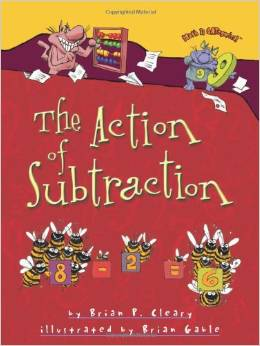# Single Digit Subtraction Word Problems

14 teachers like this lesson
Print Lesson

## Objective

SWBAT solve subtraction word problems.

#### Big Idea

Take it away! In this lesson students will solve subtraction word problems.

## Activating Strategy

10 minutes

I like to start this lesson with the book "The Action of Subtraction"If the book isn't available, there is a You Tube version as well.

I then discuss with the students different ways to subtract.  I ask for a volunteer to name a strategy used to subtract. I have the child tell a story problem that uses that strategy. I then have the other students use counters to solve the volunteer’s problem.

I then ask another volunteer to name a different subtraction strategy and tell a story problem for it. I also have the other students use counters to solve this problem.

In this lesson, children must decide which strategy to use to solve a word problem OA.A.1.  One of the common misconceptions students may have in subtraction is assuming that a key word or phrase will always suggest the same type of operation.  It is important to teach students how to think through the word problem, rather than look for key words.

## Teaching Strategies

15 minutes

Read the following problem aloud to the class.  There is also a PPT: Subtraction word problems.

Kyle had 13 hats. He gave 5 hats to Jake. How many hats does Kyle have now?

After reading the problem with the class, work through the problem together.  Ask the students:

• What do you need to find? (the number of hats Kyle has now)
• What information do you need to use? (Kyle had 13 hats. He gave 5 hats to Jake.)

I like to provide counters and Ten Frames for modeling the problem, thus providing them with the opportunity to model with math (MP4). I ask the class:

• How did you use counters and ten frames to solve the problem? (I put 13 counters in two ten frames. I took away 5 counters.  The counters that are left are the answer.)

Read the following problem to the class:

Heather has 14 crackers.  Some crackers are broken.  8 crackers are not broken. How many crackers are broken?

• What do you need to find? (the number of crackers that are broken)
• What information do you use? (Heather has 14 crackers. 8 crackers are not broken.)

I like to have a student come to the front of the class to model which strategy they would use to solve the problem.

It is important to provide multiple opportunities for students to participate in shared problem-solving activities to solve word problems. One effective way is by collaborate in small groups to develop problem-solving strategies using a variety of models such as drawings, words, and equations with symbols for the unknown numbers to find the solutions (MP4). Additionally students need the opportunity to explain, write and reflect on their problem-solving strategies.

## Independent Practice

30 minutes

I like to hand out the subtraction word problems worksheet to give students practice in reading and working through subtraction word problems.

For struggling students, I like to read the word problem to them and then have them rephrase the problem.  It's important to make sure that they include all of the important information that is needed to solve the problem.  We then make a drawing or use manipulative to solve the problem.

I had one student counting back to subtract.  I asked him to go back and check his answer by using the strategy of using addition to check subtraction.  That is a strategy that we worked on all year to help with fluency in math facts.

## Closing/Summarizing

5 minutes

To close out the lesson, I have students use pictures in their math journal to explain how to solve the following word problem:

There are 12 bananas in the tree.  8 bananas fall to the ground.  How many bananas are there now?I like to use journals as a way to close out a lesson.  It gives me a quick check to see who understood the concept and who needs more instruction.